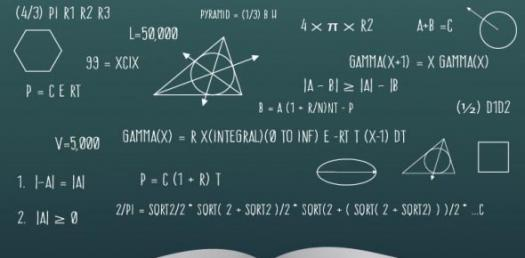# Basic Math Quiz For Beginners!

Approved & Edited by ProProfs Editorial Team
At ProProfs Quizzes, our dedicated in-house team of experts takes pride in their work. With a sharp eye for detail, they meticulously review each quiz. This ensures that every quiz, taken by over 100 million users, meets our standards of accuracy, clarity, and engagement.
| Written by Mujtaba
M
Mujtaba
Community Contributor
Quizzes Created: 1 | Total Attempts: 545
Questions: 5 | Attempts: 545SettingsMost people have the misconception that math is a difficult subject to understand, and this is a misconception that they can beat if they did some practice questions after every class. Do you think that you can handle this basic math quiz for beginners? Give it a shot and get to see how excellent your skills are.

• 1.

### What is 34 + 9 + 0 - 9 =

• A.

35

• B.

34

• C.

75

• D.

43

• E.

32

B. 34
Explanation
The given equation is 34 + 9 + 0 - 9. When we add 34 and 9, we get 43. Since we are subtracting 9 from this sum, the final result is 34.

Rate this question:

• 2.

### 25% of 60 is ________  .

15
Explanation
25% of 60 can be calculated by multiplying 60 by 0.25. This gives us 15, which is the answer.

Rate this question:

• 3.

### 45 + 3 + 2 + 22 =

• A.

12

• B.

72

• C.

72.999

• D.

73

• E.

727

B. 72
Explanation
The correct answer is 72 because when you add 45, 3, 2, and 22 together, you get a total of 72.

Rate this question:

• 4.

### 25% of 200 is _____.

• A.

34

• B.

321

• C.

50

• D.

234

• E.

23

C. 50
Explanation
25% of 200 can be calculated by multiplying 200 by 0.25. This gives us 50, which is the correct answer.

Rate this question:

• 5.

### 34 + 9 + m = 45 whaty is mBack to top